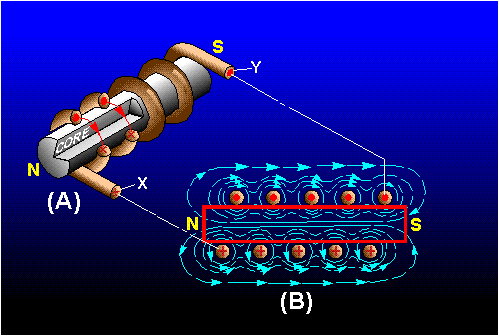Magnetic Field of a CoilCustom SearchMAGNETIC FIELD OF A COIL Figure 1-3(A) illustrates that the magnetic field around a current-carrying wire exists at all points along the wire. Figure 1-5 illustrates that when a straight wire is wound around a core, it forms a coil and that the magnetic field about the core assumes a different shape. Figure 1-5(A) is actually a partial cutaway view showing the construction of a simple coil. Figure 1-5(B) shows a cross-sectional view of the same coil. Notice that the two ends of the coil are identified as X and Y. Figure 1-5. - Magnetic field produced by a current-carrying coil.When current is passed through the coil, the magnetic field about each turn of wire links with the fields of the adjacent turns. (See figure 1-4(A)). The combined influence of all the turns produces a two-pole field similar to that of a simple bar magnet. One end of the coil is a north pole and the other end is a south pole. Polarity of an Electromagnetic Coil Figure 1-2 shows that the direction of the magnetic field around a straight wire depends on the direction of current in that wire. Thus, a reversal of current in a wire causes a reversal in the direction of the magnetic field that is produced. It follows that a reversal of the current in a coil also causes a reversal of the two-pole magnetic field about the coil. When the direction of the current in a coil is known, you can determine the magnetic polarity of the coil by using the LEFT-HAND RULE FOR COILS. This rule, illustrated in figure 1-6, is stated as follows: Figure 1-6. - Left-hand rule for coils.Grasp the coil in your left hand, with your fingers "wrapped around" in the direction of the electron current flow. Your thumb will then point toward the north pole of the coil. Strength of an Electromagnetic Field The strength or intensity of a coil's magnetic field depends on a number of factors. The main ones are listed below and will be discussed again later. The number of turns of wire in the coil. The amount of current flowing in the coil. The ratio of the coil length to the coil width. The type of material in the core. Losses in an Electromagnetic Field When current flows in a conductor, the atoms in the conductor all line up in a definite direction, producing a magnetic field. When the direction of the current changes, the direction of the atoms' alignment also changes, causing the magnetic field to change direction. To reverse all the atoms requires that power be expended, and this power is lost. This loss of power (in the form of heat) is called HYSTERESIS LOSS. Hysteresis loss is common to all ac equipment; however, it causes few problems except in motors, generators, and transformers. When these devices are discussed later in this module, hysteresis loss will be covered in more detail. Q.12 What is the shape of the magnetic field that exists around (a) a straight conductor and (b) a coil? Q.13 What happens to the two-pole field of a coil when the current through the coil is reversed? Q.14 What rule is used to determine the polarity of a coil when the direction of the electron current flow in the coil is known? Q.15 State the rule whose purpose is described in Q14.Integrated Publishing, Inc. - A (SDVOSB) Service Disabled Veteran Owned Small Business